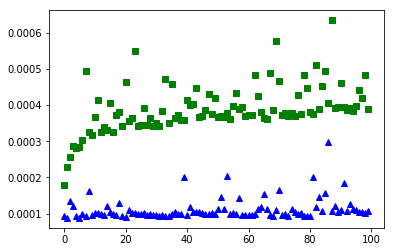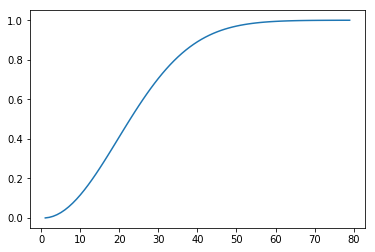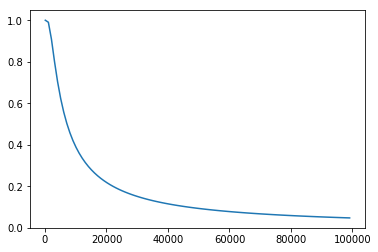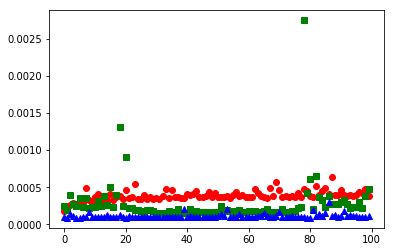# Hashtables¶

## Agenda¶

• Discussion: pros/cons of array-backed and linked structures
• Python's other built-in DS: the dict
• A naive lookup DS
• Direct lookups via Hashing
• Hashtables
• Collisions and the "Birthday problem"
• Runtime analysis & Discussion

## Discussion: pros/cons of array-backed and linked structures¶

Between the array-backed and linked list we have:

1. $O(1)$ indexing (array-backed)
2. $O(1)$ appending (array-backed & linked)
3. $O(1)$ insertion/deletion without indexing (linked)
4. $O(\log N)$ binary search, when sorted (array-backed)

## Python's other built-in DS: the dict¶

In :
import timeit

def lin_search(lst, x):
for i in range(len(lst)):
if lst[i] == x:
return i
raise ValueError(x)

def bin_search(lst, x):
# assume that lst is sorted!!!
low = 0
hi  = len(lst)
mid = (low + hi) // 2
while lst[mid] != x and low <= hi:
if lst[mid] < x:
low = mid + 1
else:
hi  = mid - 1
mid = (low + hi) // 2
if lst[mid] == x:
return mid
else:
raise ValueError(x)

def time_lin_search(size):
return timeit.timeit('lin_search(lst, random.randrange({}))'.format(size), # interpolate size into randrange
'import random ; from __main__ import lin_search ;'
'lst = [x for x in range({})]'.format(size), # interpolate size into list range
number=100)

def time_bin_search(size):
return timeit.timeit('bin_search(lst, random.randrange({}))'.format(size), # interpolate size into randrange
'import random ; from __main__ import bin_search ;'
'lst = [x for x in range({})]'.format(size), # interpolate size into list range
number=100)

def time_dict(size):
return timeit.timeit('dct[random.randrange({})]'.format(size),
'import random ; '
'dct = {{x: x for x in range({})}}'.format(size),
number=100)

lin_search_timings = [time_lin_search(n)
for n in range(10, 10000, 100)]

bin_search_timings = [time_bin_search(n)
for n in range(10, 10000, 100)]

dict_timings = [time_dict(n)
for n in range(10, 10000, 100)]

In :
%matplotlib inline
import matplotlib.pyplot as plt
# plt.plot(lin_search_timings, 'ro')
plt.plot(bin_search_timings, 'gs')
plt.plot(dict_timings, 'b^')
plt.show()## A naive lookup DS¶

In :
class LookupDS:
def __init__(self):
self.data = []

def __setitem__(self, key, value): # O(N)
for i in range(len(self.data)):
if self.data[i] == key:
self.data[i] = value # updating value
return
else:
# else clause on the loop!
# will only be run if we go through the entire for loop's range
self.data.append([key, value])

def __getitem__(self, key): # O(N)
for i in range(len(self.data)):
if self.data[i] == key:
return self.data[i]
else:
raise KeyError()

def __contains__(self, key): # O(N)
try:
_ = self[key]
return True
except:
return False

In :
l = LookupDS()
l['batman'] = 'bruce wayne'
l['superman'] = 'clark kent'
l['spiderman'] = 'peter parker'

In :
l.data

Out:
[['batman', 'bruce wayne'],
['superman', 'clark kent'],
['spiderman', 'peter parker']]
In :
l['superman'] = 'tony stark'

In :
l.data

Out:
[['batman', 'bruce wayne'],
['superman', 'tony stark'],
['spiderman', 'peter parker']]
In :
l['spiderman']

Out:
'peter parker'

## Direct lookups via Hashing¶

Hashes (a.k.a. hash codes or hash values) are simply numerical values computed for objects.

In :
hash('hello')

Out:
-6591738503317405406
In :
hash('batman')

Out:
2773662751684163654
In :
hash('batmen')

Out:
-12542849498186332
In :
[hash(s) for s in ['different', 'objects', 'have', 'very', 'different', 'hashes']]

Out:
[8320974811268224930,
-7759815270482242143,
-5081139752707418842,
-1768349861430313485,
8320974811268224930,
-5974900501332859790]
In :
[i%100 for i in range(10, 1000, 40)]

Out:
[10,
50,
90,
30,
70,
10,
50,
90,
30,
70,
10,
50,
90,
30,
70,
10,
50,
90,
30,
70,
10,
50,
90,
30,
70]
In :
[hash(s)%100 for s in ['different', 'objects', 'have', 'very', 'different', 'hashes']]

Out:
[30, 57, 58, 15, 30, 10]

## Hashtables¶

In :
class Hashtable:
def __init__(self, n_buckets=1000):
self.buckets = [None] * n_buckets

def __setitem__(self, key, val):
bucket_idx = hash(key) % len(self.buckets)
self.buckets[bucket_idx] = [key, val]

def __getitem__(self, key):
bucket_idx = hash(key) % len(self.buckets)
entry = self.buckets[bucket_idx]
if entry:
return entry
else:
raise KeyError()

def __contains__(self, key):
try:
_ = self[key]
return True
except:
return False

In :
ht = Hashtable(8)
ht['batman'] = 'bruce wayne'
ht['superman'] = 'clark kent'
ht['spiderman'] = 'peter parker'

In :
ht.buckets

Out:
[['superman', 'clark kent'],
None,
None,
None,
None,
['spiderman', 'peter parker'],
['batman', 'bruce wayne'],
None]
In :
ht = 'one'

In :
ht.buckets

Out:
[[0, 'one'],
None,
None,
None,
None,
['spiderman', 'peter parker'],
['batman', 'bruce wayne'],
None]
In :
ht['superman']

Out:
'one'

## On Collisions¶

### The "Birthday Problem"¶

Problem statement: Given $N$ people at a party, how likely is it that at least two people will have the same birthday?

In :
def birthday_p(n_people):
p_inv = 1
for n in range(365, 365-n_people, -1):
p_inv *= n / 365
return 1 - p_inv

In :
birthday_p(3)

Out:
0.008204165884781345
In :
1-(364/365*363/365)

Out:
0.008204165884781456
In :
%matplotlib inline
import matplotlib.pyplot as plt

n_people = range(1, 80)
plt.plot(n_people, [birthday_p(n) for n in n_people])
plt.show()### General collision statistics¶

Repeat the birthday problem, but with a given number of values and "buckets" that are allotted to hold them. How likely is it that two or more values will map to the same bucket?

In :
def collision_p(n_values, n_buckets):
p_inv = 1
for n in range(n_buckets, n_buckets-n_values, -1):
p_inv *= n / n_buckets
return 1 - p_inv

In :
collision_p(23, 365) # same as birthday problem, for 23 people

Out:
0.5072972343239857
In :
collision_p(10, 100)

Out:
0.37184349044470544
In :
collision_p(100, 1000)

Out:
0.9940410733677595
In :
# keeping number of values fixed at 100, but vary number of buckets: visualize probability of collision
%matplotlib inline
import matplotlib.pyplot as plt

n_buckets = range(100, 100001, 1000)
plt.plot(n_buckets, [collision_p(100, nb) for nb in n_buckets])
plt.show()In :
def avg_num_collisions(n, b):
"""Returns the expected number of collisions for n values uniformly distributed
over a hashtable of b buckets. Based on (fairly) elementary probability theory.
(Pay attention in MATH 474!)"""
return n - b + b * (1 - 1/b)**n

In :
avg_num_collisions(28, 365)

Out:
1.011442040700615
In :
avg_num_collisions(1000, 1000)

Out:
367.6954247709637
In :
avg_num_collisions(1000, 10000)

Out:
48.32893558556316

## Dealing with Collisions¶

To deal with collisions in a hashtable, we simply create a "chain" of key/value pairs for each bucket where collisions occur. The chain needs to be a data structure that supports quick insertion — natural choice: the linked list!

In :
class Hashtable:
class Node:
def __init__(self, key, val, next=None):
self.key = key
self.val = val
self.next = next

def __init__(self, n_buckets=1000):
self.buckets = [None] * n_buckets

def __setitem__(self, key, val):
bucket_idx = hash(key) % len(self.buckets)
b = self.buckets[bucket_idx]
while b:
if b.key == key:
b.val = val
return
b = b.next
else:
self.buckets[bucket_idx] = Hashtable.Node(key, val, next=self.buckets[bucket_idx])

def __getitem__(self, key):
bucket_idx = hash(key) % len(self.buckets)
b = self.buckets[bucket_idx]
while b:
if b.key == key:
return b.val
b = b.next
else:
raise KeyError()

def __contains__(self, key):
try:
_ = self[key]
return True
except:
return False

In :
ht = Hashtable(1)
ht['batman'] = 'bruce wayne'
ht['superman'] = 'clark kent'
ht['spiderman'] = 'peter parker'

In :
ht.buckets

Out:
[<__main__.Hashtable.Node at 0x10bad2eb8>]
In :
ht['batman']

Out:
'bruce wayne'
In :
ht['superman']

Out:
'clark kent'
In :
ht['spiderman']

Out:
'peter parker'
In :
def prep_ht(size):
ht = Hashtable(size*10)
for x in range(size):
ht[x] = x
return ht

def time_ht(size):
return timeit.timeit('ht[random.randrange({})]'.format(size),
'import random ; from __main__ import prep_ht ;'
'ht = prep_ht({})'.format(size),
number=100)

ht_timings = [time_ht(n)
for n in range(10, 10000, 100)]

In :
%matplotlib inline
import matplotlib.pyplot as plt
plt.plot(bin_search_timings, 'ro')
plt.plot(ht_timings, 'gs')
plt.plot(dict_timings, 'b^')
plt.show()## Loose ends¶

### Iteration¶

In :
class Hashtable(Hashtable):
def __iter__(self):
for b in self.buckets:
while b:
yield b.key
b = b.next

In :
ht = Hashtable(1)
ht['batman'] = 'bruce wayne'
ht['superman'] = 'clark kent'
ht['spiderman'] = 'peter parker'

In :
for k in ht:
print(k)

spiderman
superman
batman


### Key ordering¶

In :
ht = Hashtable()
d = {}
for x in 'apple banana cat dog elephant'.split():
d[x] = x
ht[x] = x

In :
for k in d:
print(k, '=>', d[k])

a => apple
b => banana
c => cat
d => dog
e => elephant

In :
for k in ht:
print(k, '=>', ht[k])

b => banana
e => elephant
a => apple
c => cat
d => dog


It doesn't often make sense to start with a large number of buckets, unless we know in advance that the number of keys is going to be vast — also, the user of the hashtable would typically prefer to not be bothered with implementation details (i.e., bucket count) when using the data structure.

Instead: start with a relatively small number of buckets, and if the ratio of keys to the number of buckets (known as the load factor) is above some desired threshold — which we can determine using collision probabilities — we can dynamically increase the number of buckets. This requires, however, that we rehash all keys and potentially move them into new buckets (since the hash(key) % num_buckets mapping will likely be different with more buckets).

### Other APIs¶

• FIXED __setitem__ (to update value for existing key)
• __delitem__
• keys & values (return iterators for keys and values)
• setdefault

## Runtime analysis & Discussion¶

For a hashtable with $N$ key/value entries:

• Insertion: $O(?)$
• Lookup: $O(?)$
• Deletion: $O(?)$

## Vocabulary list¶

• hashtable
• hashing and hashes
• collision
• hash buckets & chains
• birthday problem
• rehashing

Remember: a given object must always hash to the same value. This is required so that we can always map the object to the same hash bucket.

Hashcodes for collections of objects are usually computed from the hashcodes of its contents, e.g., the hash of a tuple is a function of the hashes of the objects in said tuple:

In [ ]:
hash(('two', 'strings'))


This is useful. It allows us to use a tuple, for instance, as a key for a hashtable.

However, if the collection of objects is mutable — i.e., we can alter its contents — this means that we can potentially change its hashcode.

If we were to use such a collection as a key in a hashtable, and alter the collection after it's been assigned to a particular bucket, this leads to a serious problem: the collection may now be in the wrong bucket (as it was assigned to a bucket based on its original hashcode)!

For this reason, only immutable types are, by default, hashable in Python. So while we can use integers, strings, and tuples as keys in dictionaries, lists (which are mutable) cannot be used. Indeed, Python marks built-in mutable types as "unhashable", e.g.,

In [ ]:
hash([1, 2, 3])


That said, Python does support hashing on instances of custom classes (which are mutable). This is because the default hash function implementation does not rely on the contents of instances of custom classes. E.g.,

In [ ]:
class Student:
def __init__(self, fname, lname):
self.fname = fname
self.lname = lname

In [ ]:
s = Student('John', 'Doe')
hash(s)

In [ ]:
s.fname = 'Jane'
hash(s) # same as before mutation


We can change the default behavior by providing our own hash function in __hash__, e.g.,

In [ ]:
class Student:
def __init__(self, fname, lname):
self.fname = fname
self.lname = lname

def __hash__(self):
return hash(self.fname) + hash(self.lname)

In [ ]:
s = Student('John', 'Doe')
hash(s)

In [ ]:
s.fname = 'Jane'
hash(s)
`

But be careful: instances of this class are no longer suitable for use as keys in hashtables (or dictionaries), if you intend to mutate them after using them as keys!